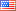Ask Your Question

# Revision history [back]

### hyphen in subscript

Sometimes, the hyphen as subscript doesn't appear : see the A_- in the fragment

pp= Graphics()
sage: pp+=text("$A_-$",(0,0),axes=False,fontsize=20)
sage: pp+=text("$B_-C_+$",(1,0),axes=False,fontsize=20)
sage: pp

Some hint ?

lg2 No.2 RevisionJohn Palmieri6169 ●18 ●56 ●137 http://www.math.washin...

### hyphen in subscript

Sometimes, the hyphen as subscript doesn't appear : see the A_- in the fragment

sage: pp=  Graphics() Graphics()
sage: pp+=text("$A_-$",(0,0),axes=False,fontsize=20) pp+=text("$A_-$",(0,0),axes=False,fontsize=20)
sage: pp+=text("$B_-C_+$",(1,0),axes=False,fontsize=20) pp+=text("$B_-C_+$",(1,0),axes=False,fontsize=20)
sage: pppp


Some hint ?

lg